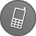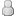• 11 Votes - 4.64 Average
• 1
• 2
• 3
• 4
• 5
11-20-2021, 02:54 AM (This post was last modified: 11-21-2021 06:02 AM by YouFeng.)Post: #376
 YouFengJunior MemberPosts: 4 Joined: Oct 2021 Reputation: 0
Code:
```// Aces Tonemap //u_setting.xyz default values (1,0,1) //Should I post here? #ifdef GL_ES precision highp float; precision highp int; #endif uniform sampler2D sampler0; varying vec2 v_texcoord0; uniform vec4 u_setting; const mat3 ACESInputMat = mat3(     0.59719, 0.07600, 0.02840,     0.35458, 0.90834, 0.13383,     0.04823, 0.01566, 0.83777 ); const mat3 ACESOutputMat = mat3(     1.60475, -0.10208, -0.00327,     -0.53108,  1.10813, -0.07276,     -0.07367, -0.00605,  1.07602 ); vec3 RRTAndODTFit(vec3 v) {     vec3 a = v * (v + 0.0245786) - 0.000090537;     vec3 b = v * (0.983729 * v + 0.4329510) + 0.238081;     return a / b; } vec3 aces_main_bakinglab(vec3 color ) {           color.x = pow(abs(color.x),u_setting.z);   color.y = pow(abs(color.y),u_setting.z);   color.z = pow(abs(color.z),u_setting.z);              color *= exp2(u_setting.y);     color = ACESInputMat * color;          color = RRTAndODTFit(color);          color = ACESOutputMat*color;          color.x = clamp(color.x,0.0,1.0);     color.y = clamp(color.y,0.0,1.0);     color.z = clamp(color.z,0.0,1.0);              color.x = pow(abs(color.x),1.0/u_setting.z);     color.y = pow(abs(color.y),1.0/u_setting.z);     color.z = pow(abs(color.z),1.0/u_setting.z);          return color; } void main() { vec3 color = texture2D(sampler0, v_texcoord0).rgb; vec3 temp_color = color; color = aces_main_bakinglab(color) * (1.0/aces_main_bakinglab(vec3(1.0,1.0,1.0))); color = mix(temp_color,color,u_setting.x);     gl_FragColor.rgb = color.rgb; }```
Code:
```//Color Temperature //Can change Game's color temperature cold or warm //u_setting.xy default value (400,1) #ifdef GL_ES precision mediump float; precision mediump int; #endif uniform sampler2D sampler0; varying vec2 v_texcoord0; uniform vec4 u_setting; vec3 ColorTemperatureToRGB(float temperatureInKelvins) {     vec3 retColor;          temperatureInKelvins = clamp(temperatureInKelvins, 1000.0, 40000.0) / 100.0;          if (temperatureInKelvins <= 66.0)     {         retColor.r = 1.0;         retColor.g = clamp((0.39008157876901960784 * log(temperatureInKelvins) - 0.63184144378862745098), 0.0, 1.0);     }     else     {         float t = temperatureInKelvins - 60.0;         retColor.r = clamp((1.29293618606274509804 * pow(t, -0.1332047592)), 0.0, 1.0);         retColor.g = clamp((1.12989086089529411765 * pow(t, -0.0755148492)), 0.0, 1.0);     }          if (temperatureInKelvins >= 66.0)         retColor.b = 1.0;     else if(temperatureInKelvins <= 19.0)         retColor.b = 0.0;     else         retColor.b = clamp((0.54320678911019607843 * log(temperatureInKelvins - 10.0) - 1.19625408914), 0.0, 1.0);     return retColor; } vec3 RGBtoHCV(vec3 RGB) {     // Based on work by Sam Hocevar and Emil Persson     vec4 P = (RGB.g < RGB.b) ? vec4(RGB.bg, -1.0, 2.0/3.0) : vec4(RGB.gb, 0.0, -1.0/3.0);     vec4 Q = (RGB.r < P.x) ? vec4(P.xyw, RGB.r) : vec4(RGB.r, P.yzx);     float C = Q.x - min(Q.w, Q.y);     float H = abs((Q.w - Q.y) / (6.0 * C ) + Q.z);     return vec3(H, C, Q.x); } vec3 RGBtoHSL(vec3 RGB) {     vec3 HCV = RGBtoHCV(RGB);     float L = HCV.z - HCV.y * 0.5;     float S = HCV.y / (1.0 - abs(L * 2.0 - 1.0) );     return vec3(HCV.x, S, L); } vec3 HUEtoRGB(float H) {     float R = abs(H * 6.0 - 3.0) - 1.0;     float G = 2.0 - abs(H * 6.0 - 2.0);     float B = 2.0 - abs(H * 6.0 - 4.0);     R = clamp(R,0.0,1.0);     G = clamp(G,0.0,1.0);     B = clamp(B,0.0,1.0);     return vec3(R,G,B); } vec3 HSLtoRGB(in vec3 HSL) {     vec3 RGB = HUEtoRGB(HSL.x);     float C = (1.0 - abs(2.0 * HSL.z - 1.0)) * HSL.y;     return (RGB - 0.5) * C + vec3(HSL.z); } void main() {         float tmp_x = (u_setting.x+1.0)*100.0;         vec3 color = texture2D(sampler0, v_texcoord0).xyz;                 vec3 kColor    = ColorTemperatureToRGB( tmp_x);         vec3 oLum      = RGBtoHSL( color.xyz );         vec3 tmp = color.xyz * kColor.xyz;         vec3 blended   = mix( color.xyz,tmp.xyz,u_setting.y);         vec3 resHSV    = RGBtoHSL( blended.xyz );         vec3 resRGB    = HSLtoRGB( vec3( resHSV.xy, oLum.z ));         color.xyz        = mix( blended.xyz, resRGB.xyz, 1.0 );         gl_FragColor.rgb = color;          }```

This is two I usually use in gta vcs

I can use pc's reshade to get game's depth buffer memory address to CE.But I don't know how to use code to operate it, damn.I guess I have to give up....

Such as far plane use high sharpening, near is low.It is the basic....But I can't even achieve.

Attached File(s) Thumbnail(s)« Next Oldest | Next Newest »